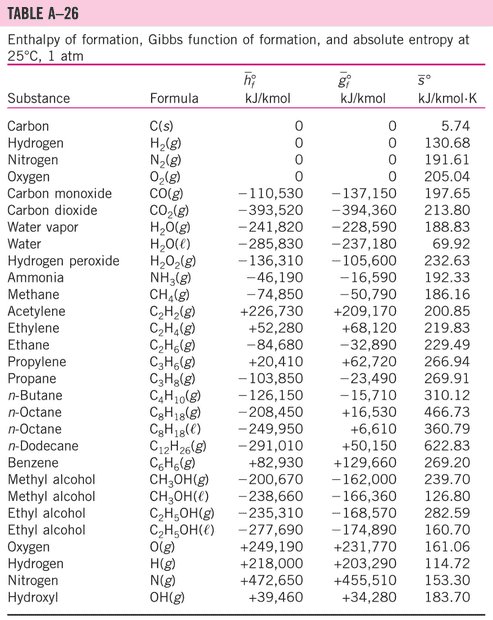# Vaporization latent heat vs boiling point

Gold Member
Homework Statement:
If the vaporization latent heat of Ethlyl alcohol under the standard conditions is 38.56 kJ/mol, estimate the boiling point of Ethlyl alcohol (in °C) according to the A26.
Relevant Equations:
vaporization latent heat = Hf-Hg = Hfg
delta G = delta H - T delta Sdelta G = delta H - T delta S
-235,310- (-277,690) = -38.56e3- T (282.59-160.70)
T = -664K

I am not sure if my concept is correct. May anyone help a little bit on that please?
thank you

Last edited:

haruspex